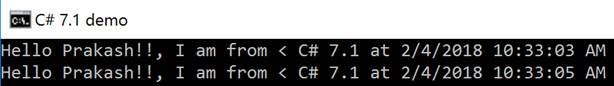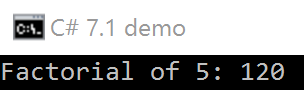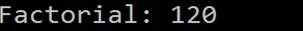# Async Main In C# 7.1

Hello folks!

I am here to present the series related to C# 7.1's new features. In the first part, we will be going through one of the important features called async main.

async main

Starting with C# 7.1, the main function that is the entry point of the application can have async. Before C# 7.1, the main function could have a return type as either void or int; however now, it also supports Task and Task<int>.

 Main overrides (Before C# 7.1) Main overrides (In C# 7.1) static void Main();   static int Main();   static void Main(string[] args);   static int Main(string[] args); static void Main();   static int Main();   static void Main(string[] args);   static int Main(string[] args);   static Task Main();   static Task < int > Main();   static Task Main(string[] args);   static Task < int > Main(string[] args);

Let’s take a few examples to understand more.

Before C# 7.1, when you wanted to call async method from Main, you needed to add some boilerplate code but now, C# compiler does it for you and, in turn, enforces crisp coding by adding the required code automatically.

Let’s try to understand this by a simple example as following.

Before C# 7.1

2. using static System.Console;
3.
4. namespace CSharp7PlusDemo
5. {
6.     class Program
7.     {
8.         static void Main(string[] args)
9.         {
10.             Title = "C# 7.1 demo";
11.             WriteLine(\$"Hello Prakash!!, I am from < C# 7.1 at {System.DateTime.Now}");
13.             WriteLine(\$"Hello Prakash!!, I am from < C# 7.1 at {System.DateTime.Now}");
14.         }
15.     }
16. }
OutputIn C# 7.1

2. using static System.Console;
3.
4. namespace CSharp7PlusDemo
5. {
6.     class Program
7.     {
8.         static async Task Main(string[] args)
9.         {
10.            WriteLine(\$"Hello Prakash!!, I am from C# 7.1 at {System.DateTime.Now}");
12.            WriteLine(\$"Hello Prakash!!, I am from C# 7.1 at {System.DateTime.Now}");
13.         }
14.     }
15. }
OutputAs you can see that Task.Delay is adding 2 seconds. Now, C# 7.1 syntax is crispier and easy to use.

So we just looked at how we could use the Task with Main. Now, let’s take another example where we will demonstrate the use of Task<int>.

Here, we will call another async method (FactorialAsync) from Main.

2. using static System.Console;
3.
4. namespace CSharp7PlusDemo
5. {
6.     class Program
7.     {
8.         static async Task<int> Main(string[] args)
9.         {
10.             Title = "C# 7.1 demo";
11.             var number = 5;
12.             WriteLine(\$"Factorial of {number}: {await FactorialAsync(number)}");
13.             return 0;
14.         }
15.
16.         private static Task<int> FactorialAsync(int n)
17.         {
19.
20.             int Cal(int i)  //Local function
21.             {
22.                 if (i == 1)
23.                 {
24.                     return 1;
25.                 }
26.                 else
27.                 {
28.                     return i * Cal(i - 1);
29.                 }
30.
31.             }
32.          }
33.      }
34. }
OutputYou can also see that in the above example, we have used a Local function that is the feature of C# 7.0. Moreover, we can also use expression-bodied method (introduced as part of C# 6.0) to call the async method directly from Main, as following.

1. static async Task Main(string[] args) => WriteLine(\$"Factorial: {await FactorialAsync(5)}");
OutputConclusion

In this article, we have looked at one of the quite useful features “async main”, introduced as part of C# 7.1. We have seen how to use Task and Task<int> with Main. On the sideline, we have also seen the use of Local function and expression bodied method.